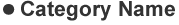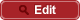• HOME
• RIKEN-QHP
• Measurement of the branching fraction and time-dependent $CP$ asymmetry for $B^0 \to J/\psi \pi^0$ decays

##PrePrint No. RIKEN-QHP-399 B. Pal et al., (Belle Collaboration) including M. Takizawa Measurement of the branching fraction and time-dependent $CP$ asymmetry for $B^0 \to J/\psi \pi^0$ decays arXiv: 1810.01356  (org) Phys. Rev. D in press 2018.10